# OECD Gender wage gap data analysis 2 - Statistical Inference with Infer package, mean difference, calculation p-value with simulation based methodUnsplashJames Wainscoatが撮影した写真

This post is following of the above post.

In the previous post I see mean of EMPLOYEE is 17.9 and mean of SELFEMPLOYED is 30.6.

Let's see whether the difference is statistically significant or not.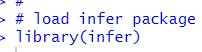Before doing statistical inference, let's do visualization.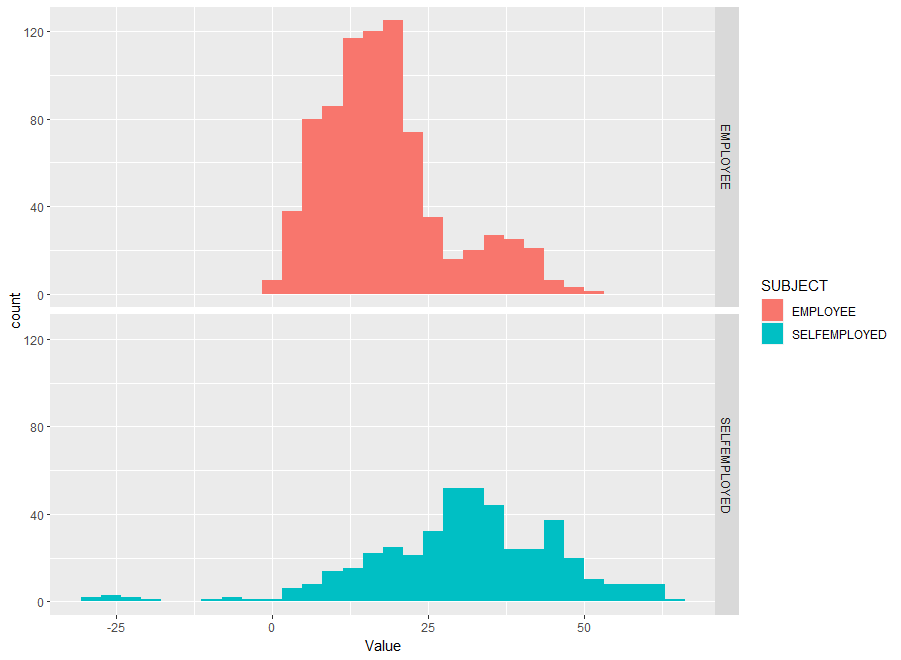I see SELFEMPLOYED Value is wider spreaded than EMPLOYEE Value.

Now, let's start statistial inference.
I will follow the methodology of B Inference Examples | Statistical Inference via Data Science (moderndive.com)

I calculate observed statistics, difference of mean, SELEMPLOYED mean - EMPLOYEE mean.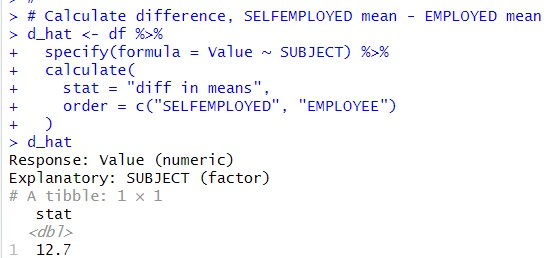So, 12.7 is the difference.

Then, I make null distribution of the difference.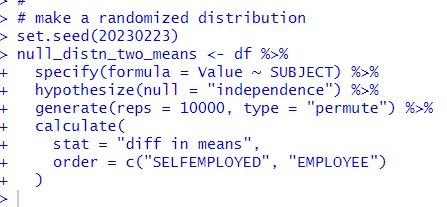Now, I can do visualization for null distribution using visualize() function.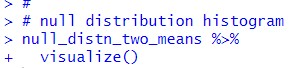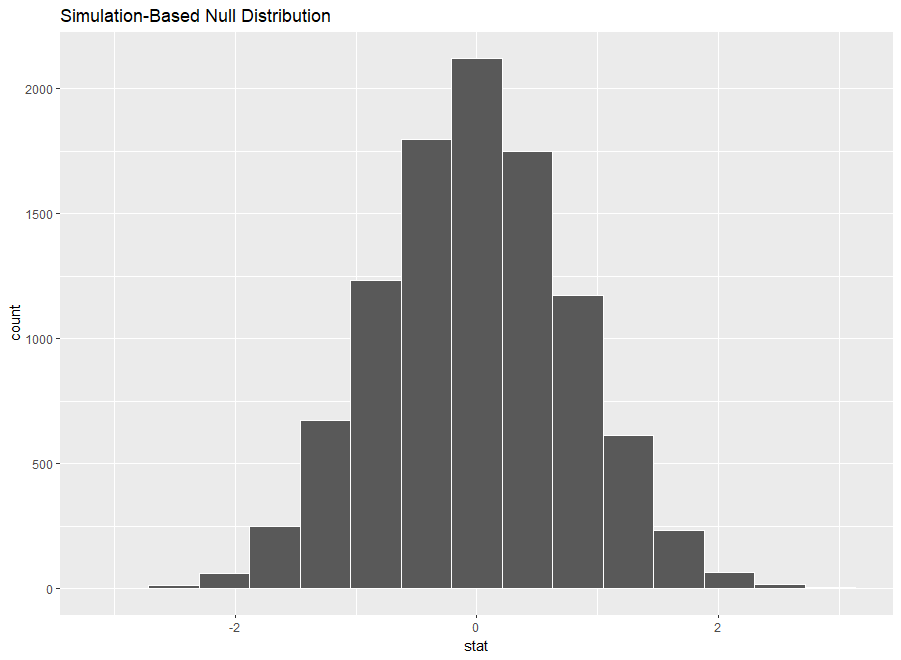I see the center of stat is 0.

Let's see p-value on the above histogram.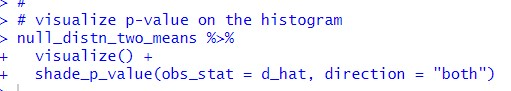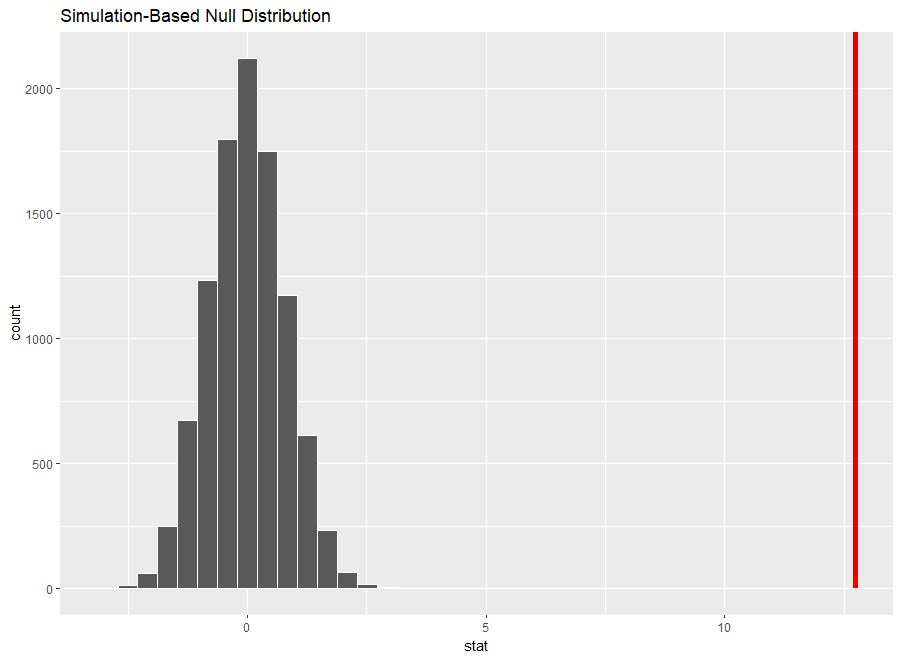I see the red vertical line is far away from the null distribution, it means p-value is very very small.

Let's calculate p-value.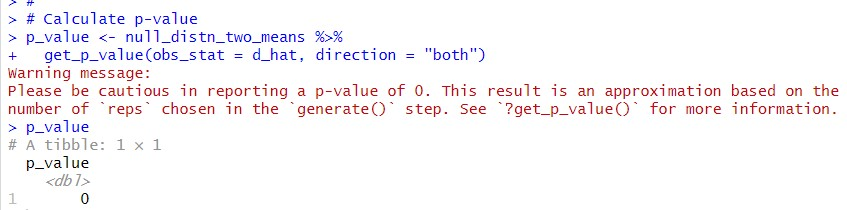Calculate p-value is 0. So I can say the difference of mean between SELFEMPLOYED and EMPLOYEE is statistically significant.

That's it thank you!
Thank you Statistical Inference via Data Science (moderndive.com) !

Next post is